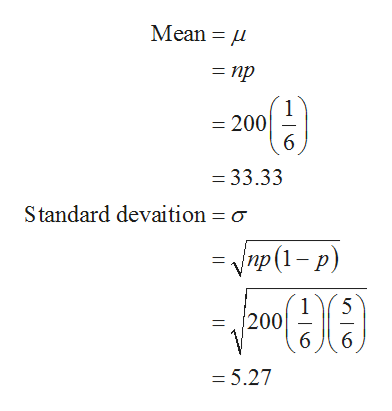# Find the probability that in 200 tosses of a fair die, we will obtain at least 40 fives

Question
349 views

Find the probability that in 200 tosses of a fair die, we will obtain at least 40 fives

check_circle

Step 1

Binomial approximation:

The normal distribution can be used as an approximation to the binomial distribution, under certain circumstances, namely:

• If X ~ B (n, p) and if n is large and/or p is close to ½, then X is approximately N (np, npq)

(where q = 1 - p).

Continuity Correction:

The binomial and Poisson distributions are discrete random variables, whereas the normal distribution is continuous. We need to take this into account when we are using the normal distribution to approximate a binomial or Poisson using a continuity correction.

Continuity correction factor table:

If   P(X=n) use   P(n – 0.5 < X < n + 0.5)
If   P(X > n) use   P(X > n + 0.5)
If   P(X ≤ n) use    P(X < n + 0.5)
If    P (X < n) use   P(X < n – 0.5)
If    P(X ≥ n) use   P(X > n – 0.5)

z-score:

If a random variable X has a distribution with mean µ and standard deviation σ, then the z-score is defined as,

Step 2

Computing the probability that at least 26 people experience flu symptoms:

Let the random variable X represents the number of fives obtained.

The probability getting a 5 when a die is tossed is 1/6.

The die is tossed 200 times.

That is, the random variable X follows binomial dis...help_outlineImage TranscriptioncloseMean = np 1 =200 6 - 33.33 Standard devaition = o -Vnp (1- p) 5 1 200 6 1 6 - 5.27 fullscreen

### Want to see the full answer?

See Solution

#### Want to see this answer and more?

Solutions are written by subject experts who are available 24/7. Questions are typically answered within 1 hour.*

See Solution
*Response times may vary by subject and question.
Tagged in

### Other